# 978-0123869449 Chapter 6 Part 3

Document Type
Homework Help
Book Title
Authors
Michael F. ModestCHAPTER 6 201
0.51
0.51×0.5199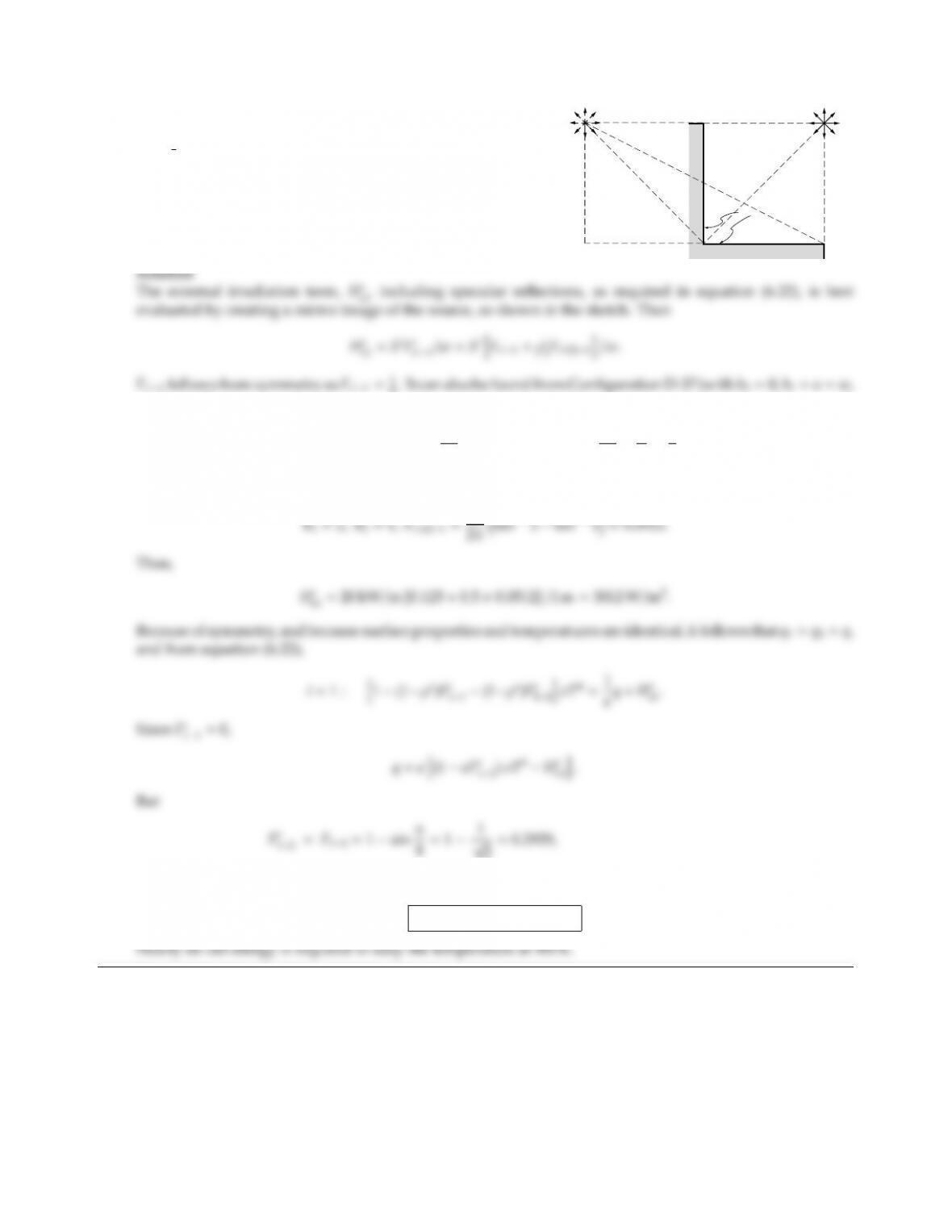6.26
w
w
w
w
T,
S´
S´
(2)
A2
A1
An infinitely long corner of characteristic length w=1 m is
a gray, diuse emitter and purely specular reflector with ǫ=
ρs=1
2. The entire corner is kept at a constant temperature
T=500 K, and is irradiated externally by a line source of
strength S=20 kW/m, located a distance waway from both
sides of the corner, as shown in the sketch. What is the total
heat flux Q(per m length) to be supplied or extracted from
the corner to keep the temperature at 500 K?
8. It can also be found from Configuration D-37 (with b2=0, b1=a=w,
and rsmall) as
B1=1,B2=0,Fs1=1
2πtan11tan10=1
2π×π
4=1
8.
Similarly, Fs(2)1is found also from Appendix D Configuration 37 (with b2=a=w,b1=2w) as
q=0.5×h(10.50.2929) 5.670 ×108×50043012i=6.3 W/m2,
Q=q×2w=12.6 W/m.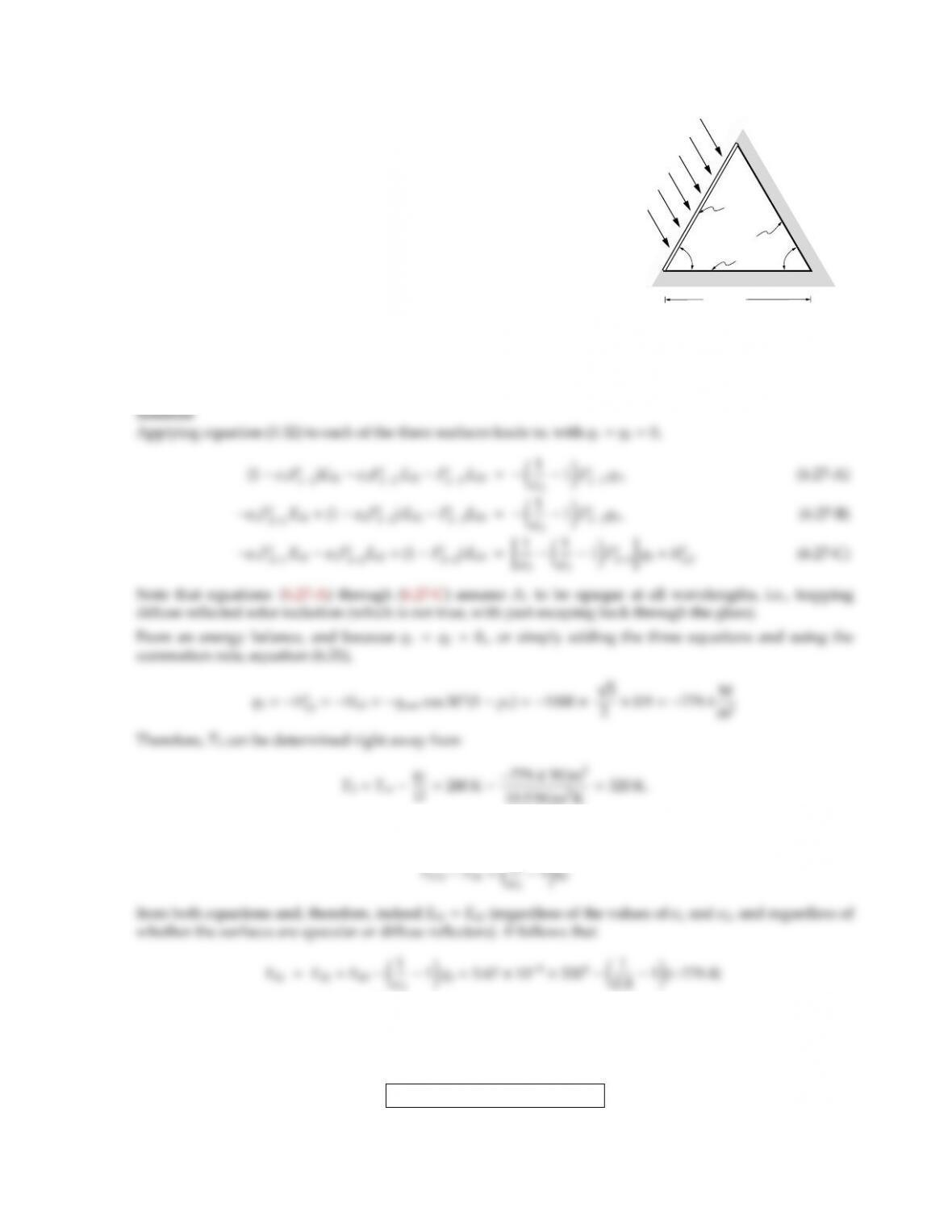CHAPTER 6 203
6.27
60°
A2
A1
A3
60°
L = 1 m
ground
qsun
A long greenhouse has the cross-section of an equilateral triangle as
shown. The side exposed to the sun consists of a thin sheet of glass
(A1) with reflectance ρ1=0.1. The glass may be assumed perfectly
inside the greenhouse. The other side wall (A2) is opaque with emittance
ǫ2=0.2, while the floor (A3) has ǫ3=0.8. Both walls (A1and A2) are
specular reflectors, while the floor reflects diusely. For simplicity, you
may assume surfaces A1and A2to be perfectly insulated, while the floor
loses heat to the ground according to
q3,conduction =U(T3T)
where T=280 K is the temperature of the ground, and U=19.5W/m2K
is an overall heat transfer coecient.
Determine the temperatures of all three surfaces for the case that the sun shines onto the greenhouse with
strength qsun =1000 W/m2in a direction parallel to surface A2.
If Eb1=Eb2, then with 1 ǫ1Fs
11ǫ2Fs
12=Fs
13and 1 ǫ1Fs
21ǫ2Fs
22=Fs
23we get
=789.4 W/m2
or
T1=T2=789.4/5.67 ×1081/4=343.5 K.
T1=T2=343.5 K,T3=320 K.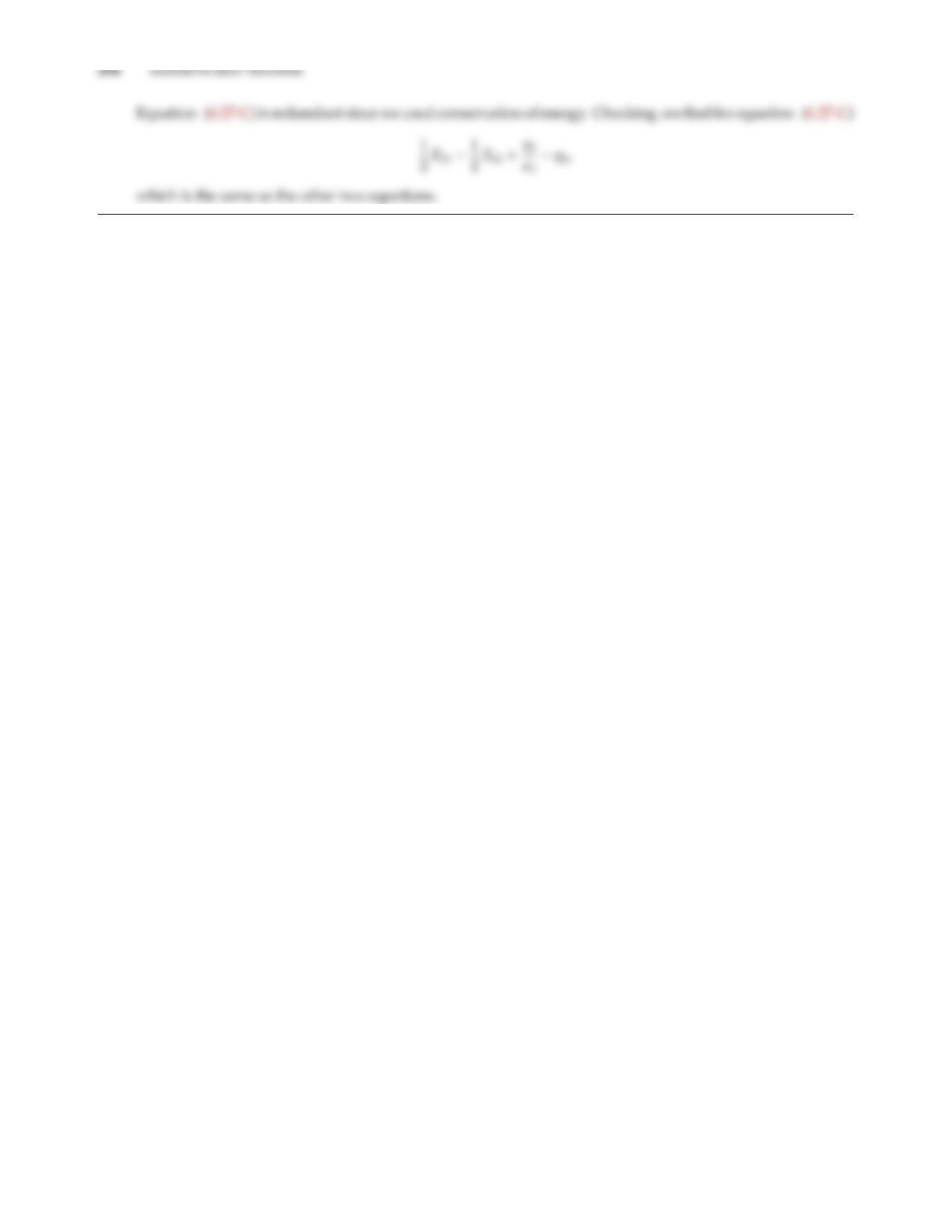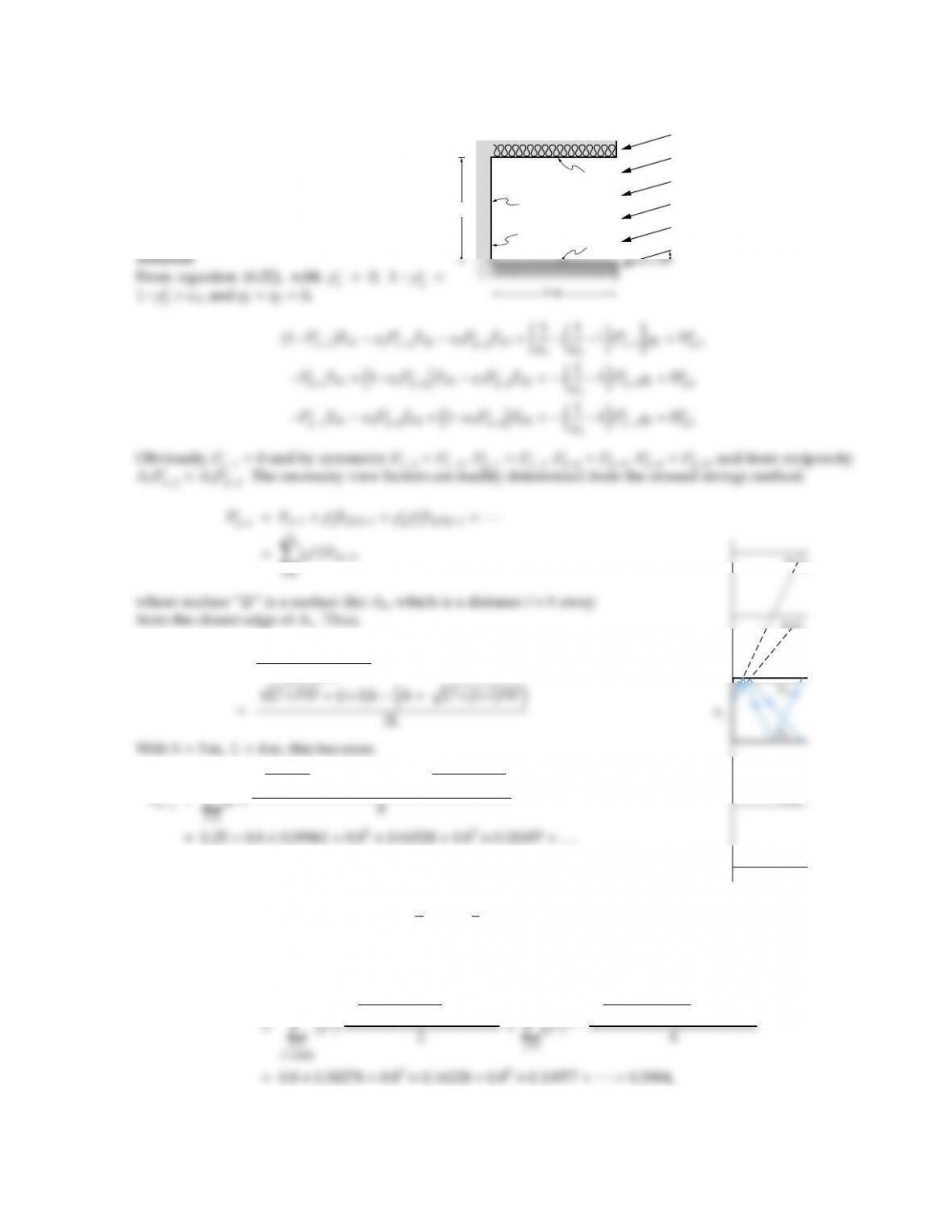CHAPTER 6 205
6.29 Consider the solar collector shown. The collec-
tor plate is gray and diuse, while the insulated
guard plates are gray and specularly reflecting.
Sun strikes the cavity at an angle α(α < 45).
How much heat is collected? Compare with a
collector without guard plates. For what val-
ues of αis your theory valid?
3 = 0.2
1 = 0.9
T1 = 400 K
2 = 0.2
3 m
qsun = 1250 W/m2
Insulated
F2i1=diagonals sides
2×base
Fs
X
(ρs)i16+9i2+3(i+1) h3i+p16+9(i+1)2i
=0.3860,
A3(23)
A3(2)
A2(32)
and
Fs
12=L
hFs
21=4
3×0.3860 =0.5147.
Similarly,
Fs
22=ρsF2(3)2+(ρs)3F2(323)2+···
X
X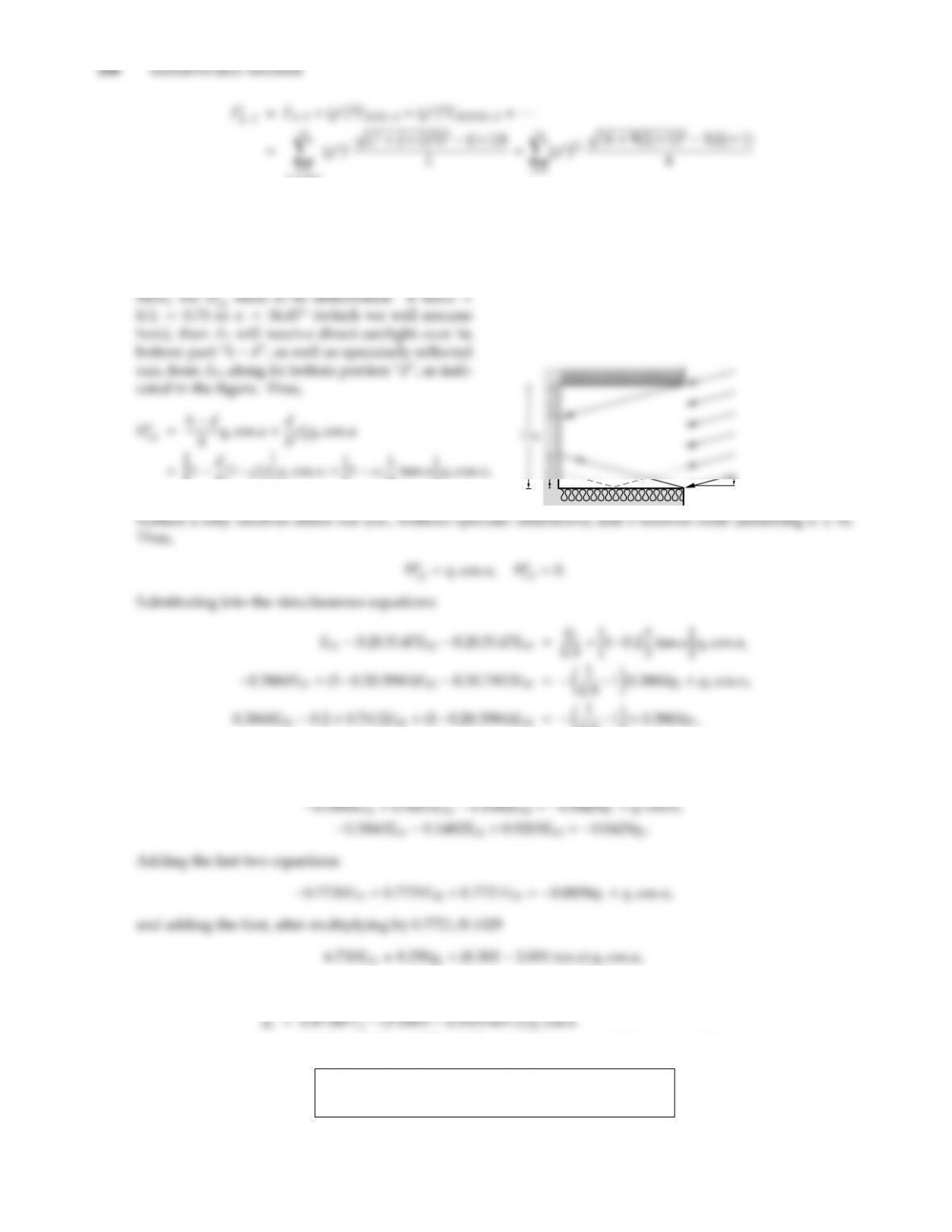(ieven)
=0.5+0.82×0.21221 +0.84×0.13104 +··· =0.7412.
One of these could also have been found using the summation rule, equation (6.21),
2Fs
21+ǫ2Fs
22+ǫ3Fs
23=20.3860+0.2(0.3984+0.7412) =0.9999.
h(1ρs
2)#qscos α=1ǫ2
htan αqscos α,
α
since d=Ltan α.
0.81×0.3860q1,
or
Eb10.1029Eb20.1029Eb3=1.1111q1+(10.2667 tan α)qscos α,
or
=0.8158 ×5.670 ×108×4004(1.0305 cos α0.2425 sin α)×1250
q1=(1288 cos α+303 sin α+1184) W/m2,
q1,max =q1(α=0)=1288 +1184 =104 W/m2.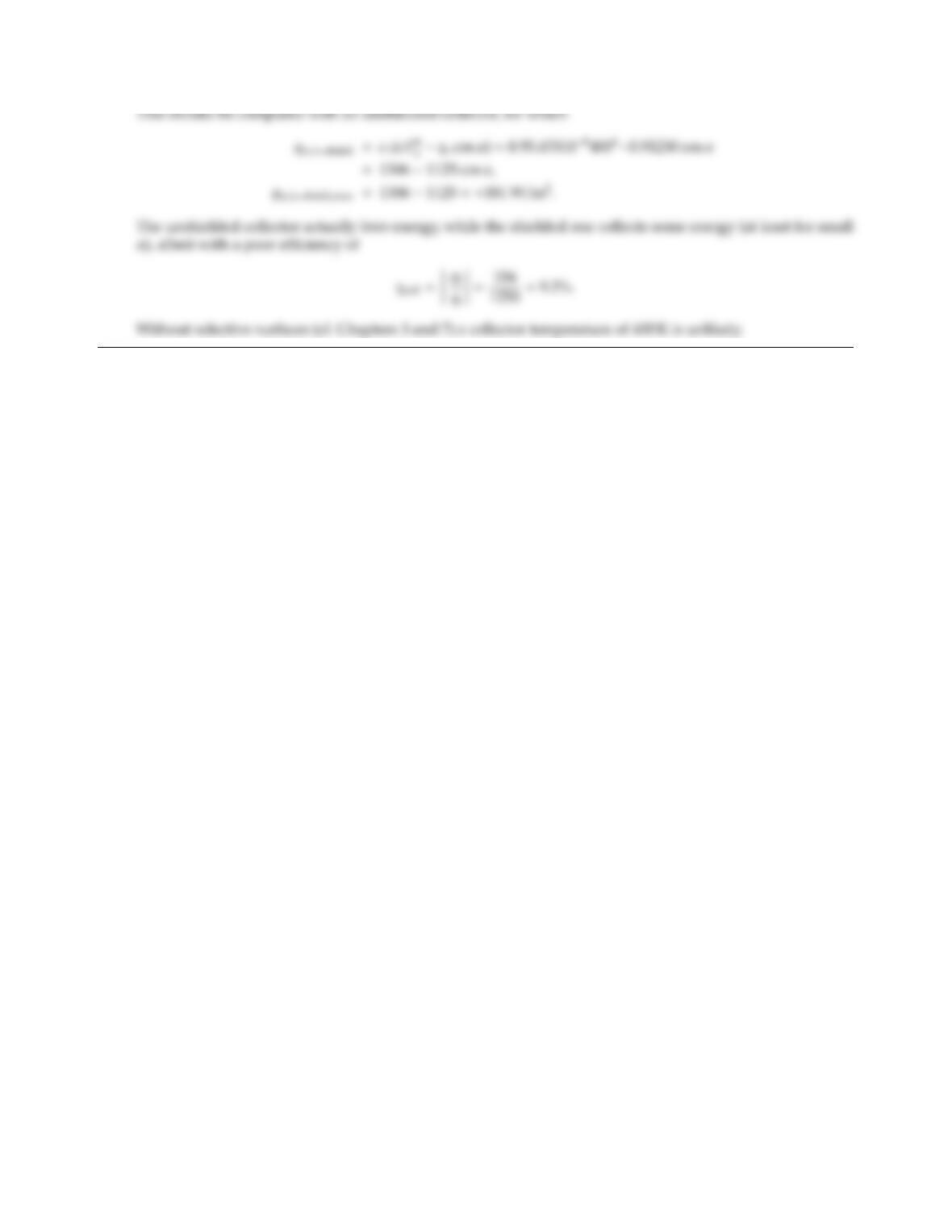CHAPTER 6 207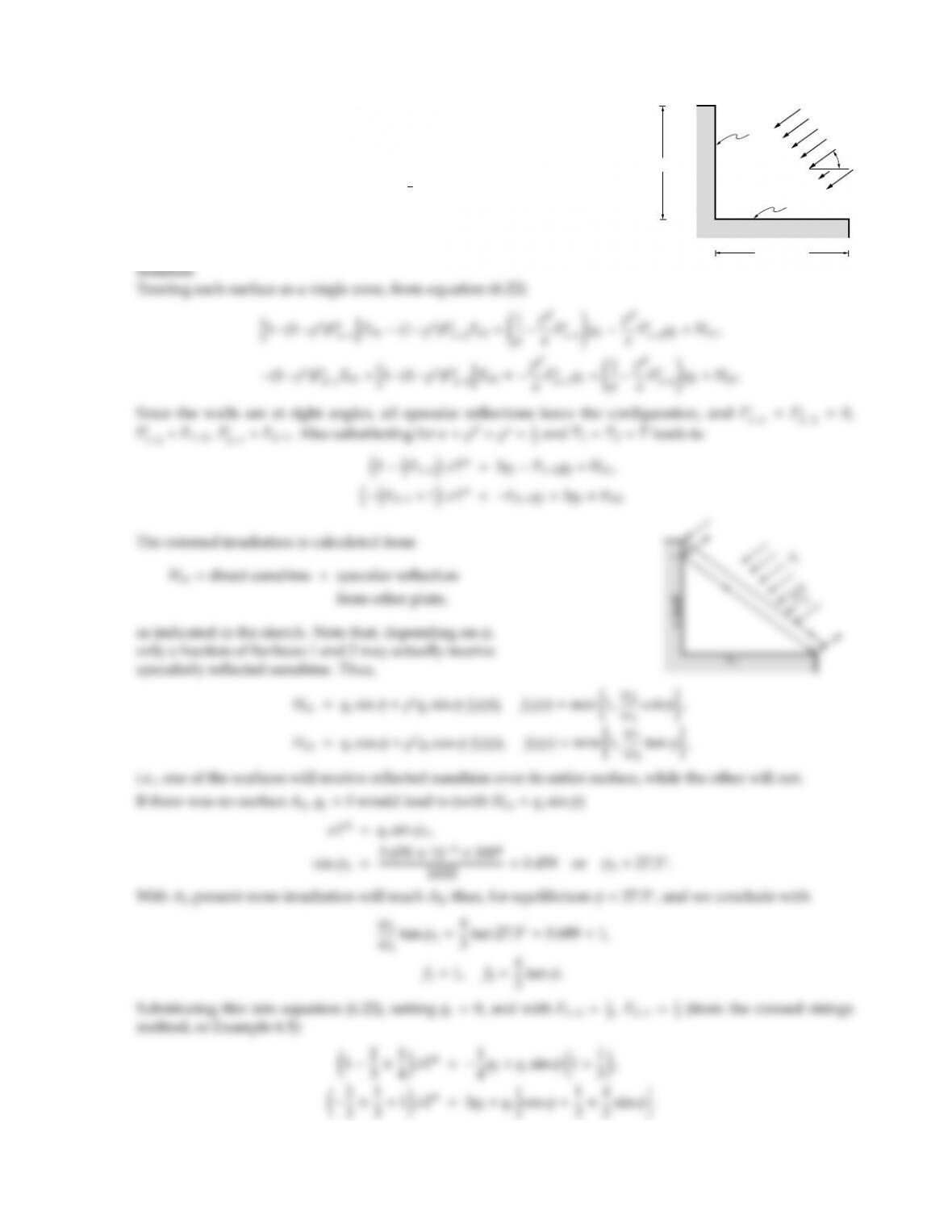6.30
w1 = 4 cm
qs
A2
A1
w2 = 3 cm
φ
A rectangular cavity as shown is irradiated by a parallel-light source
of strength qs=1000 W/m2. The entire cavity is held at constant
temperature T=300 K and is coated with a gray material whose
reflectance may be idealized to consist of purely diuse and specular
components, such that ǫ=ρd=ρs=1
3. How must the cavity be
oriented toward the light source (i.e., what is φ?) so that there is no
net heat flux on surface A1?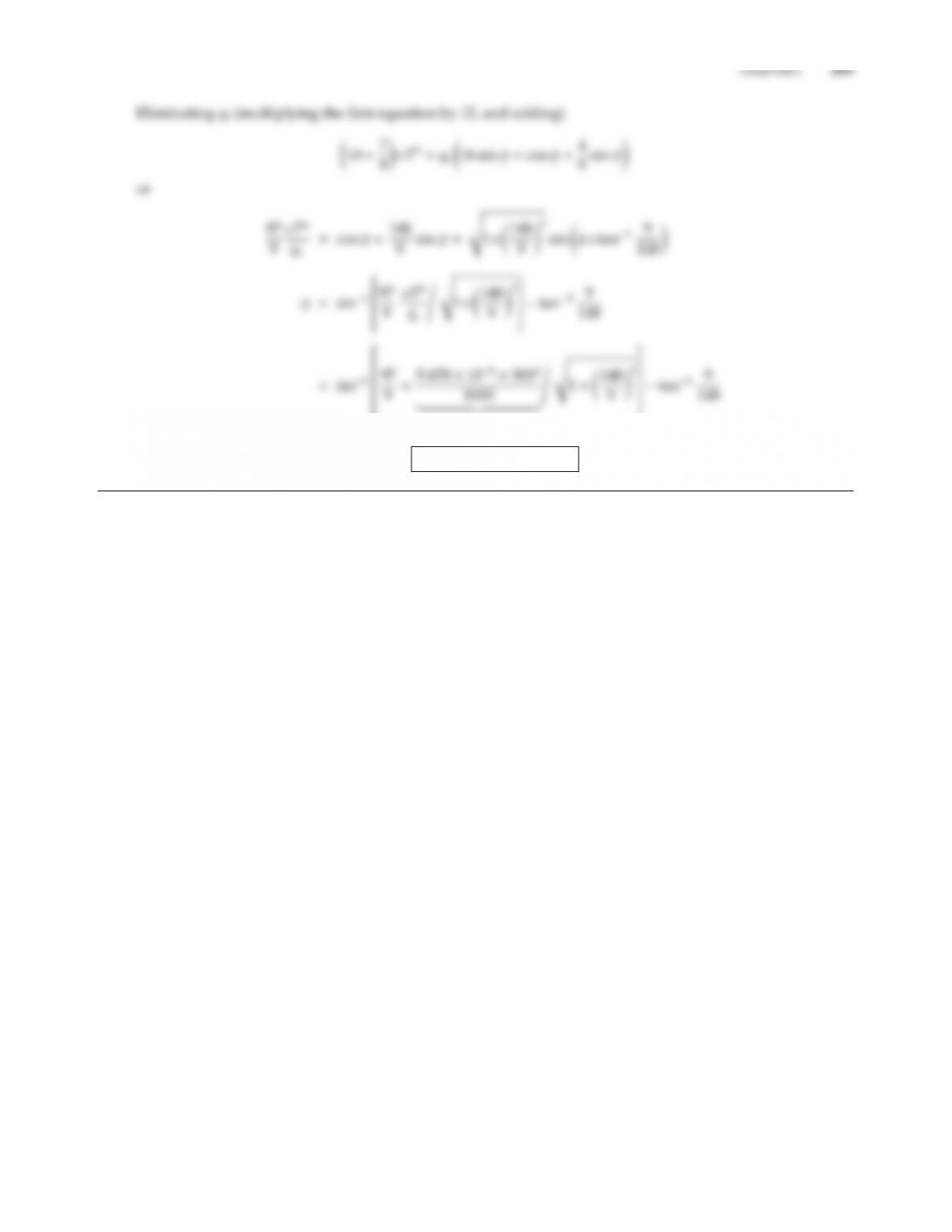0.459
φ=17.53.5=14.0.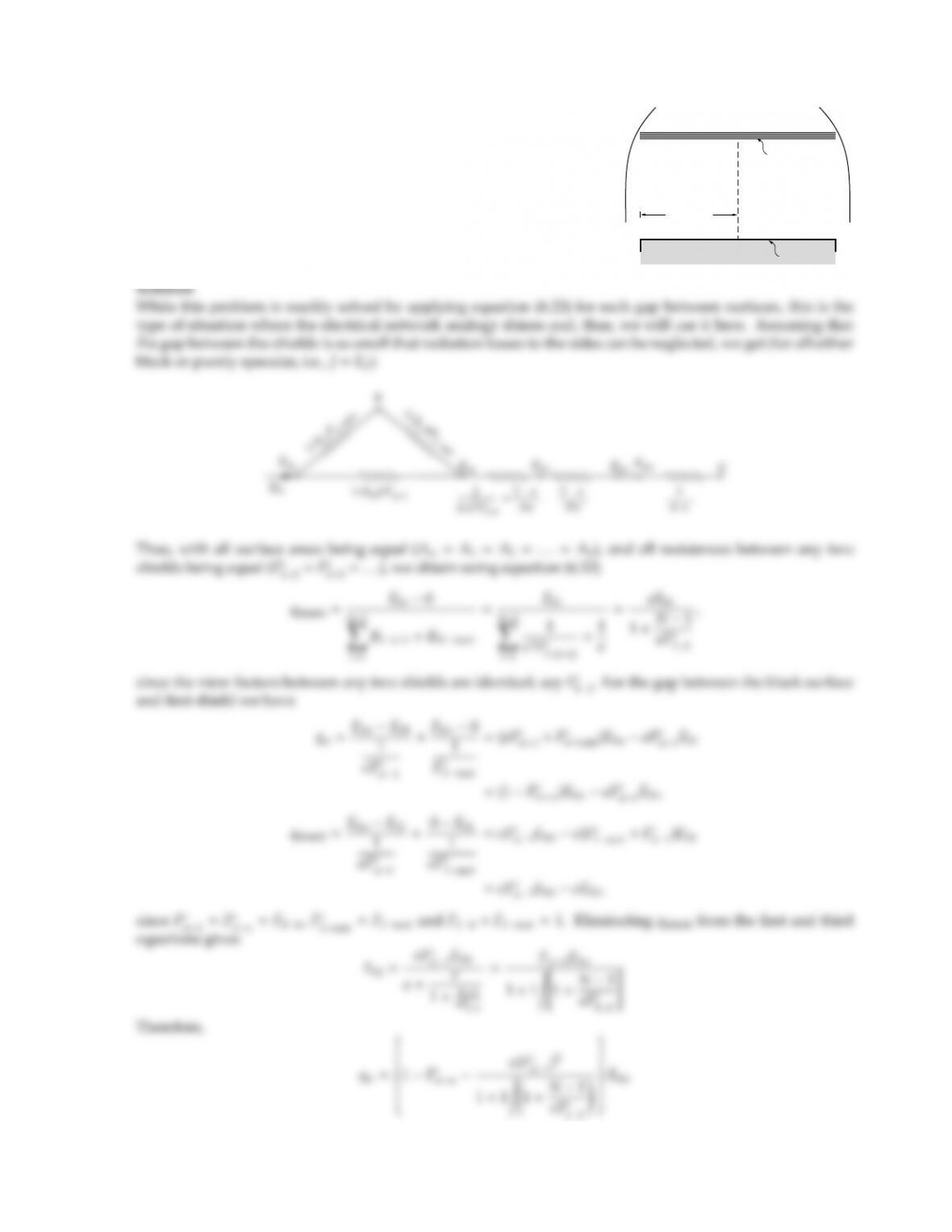6.31
h = 1 m
w = 2 m
Tw, w = 1
0 K
= 0.1
0 K
N shields,
Reconsider the spacecraft of Problem 6.10. To decrease the heat
loss from Surface 2 the specularly reflecting shield 1 is replaced
by an array of Nshields (parallel to each other and very closely
spaced), of the same dimensions as the black surface and made
of the original, specularly reflecting shield material with emittance
ǫ=0.1. Determine the net heat loss from the black plate as a function
of shield number N.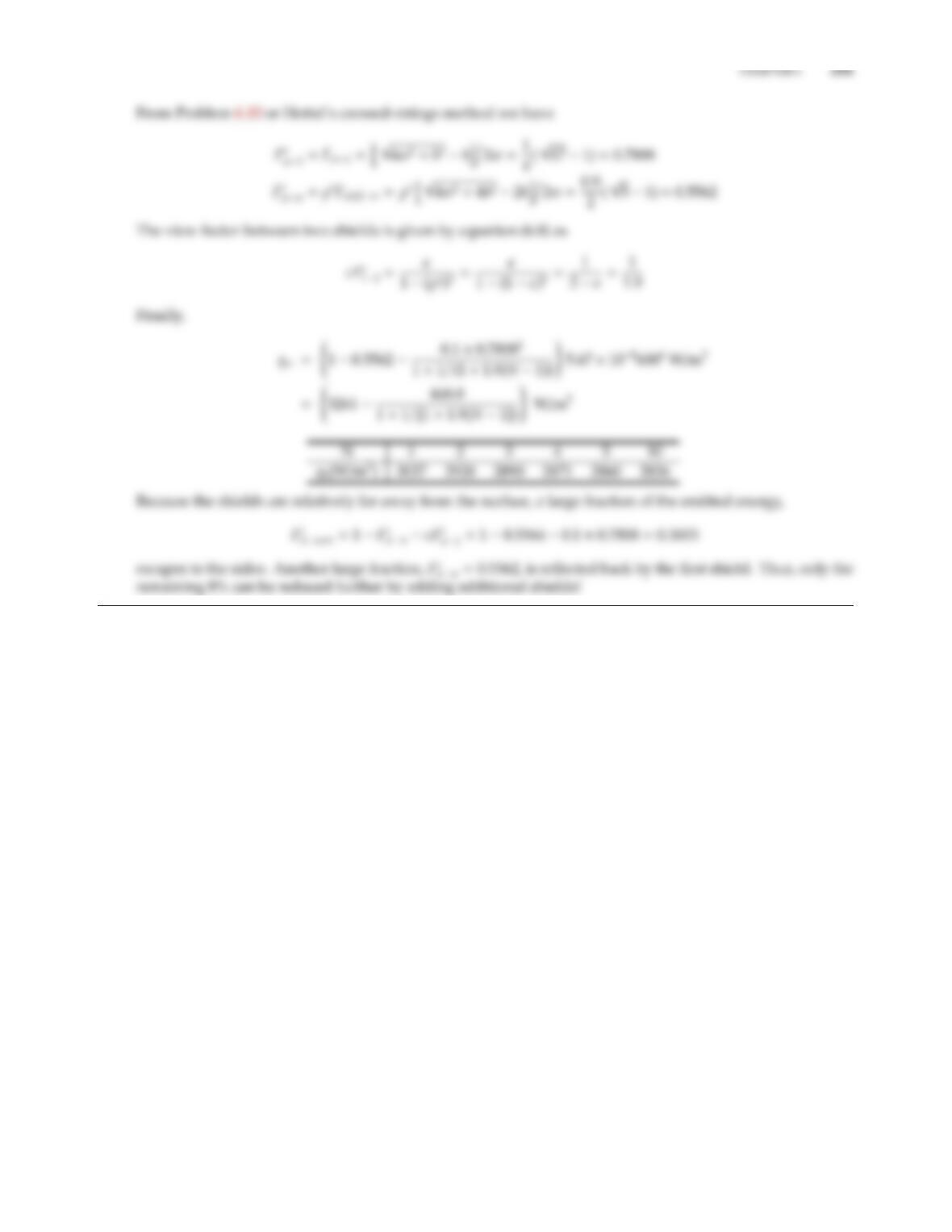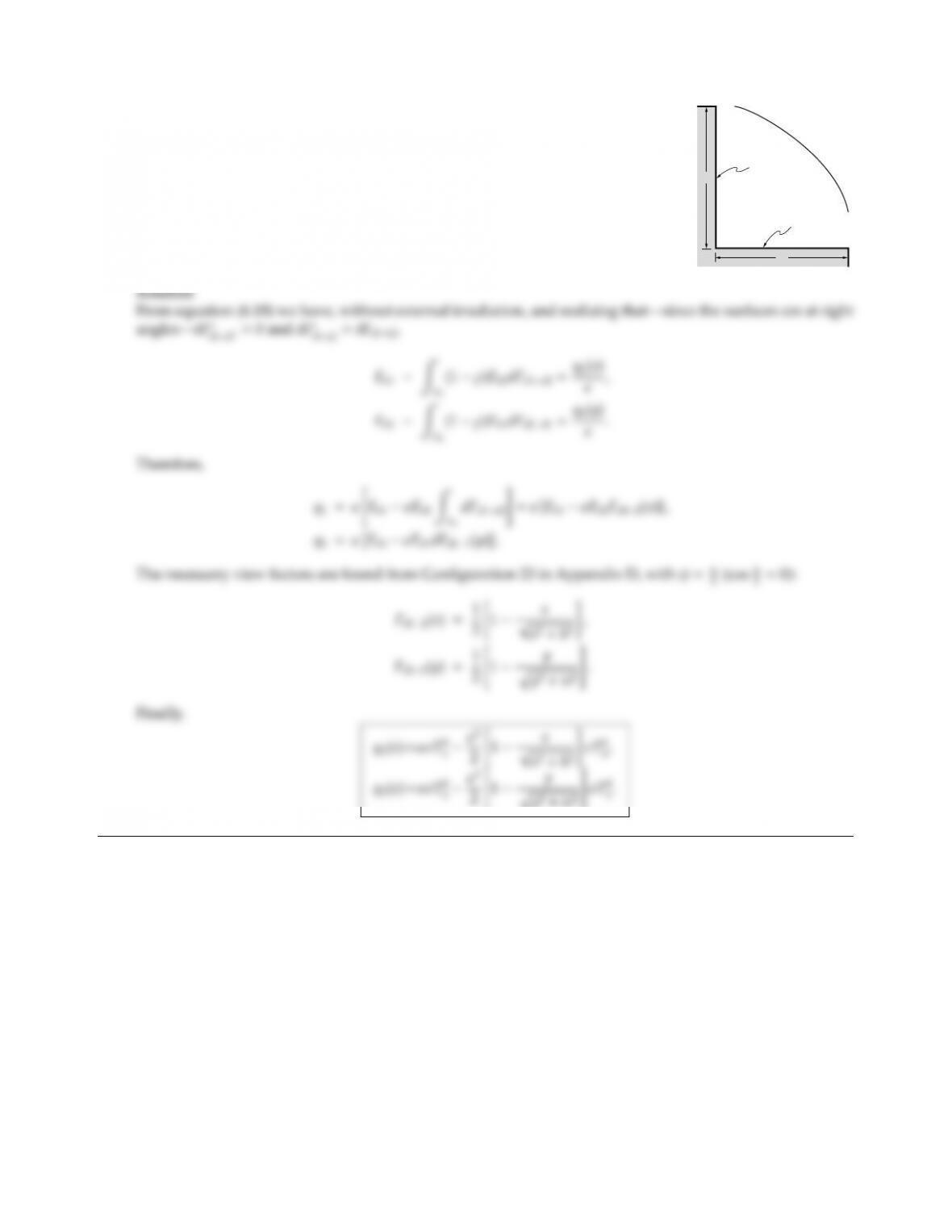6.34
h
w
A2: T2,
A1: T1,
0K
An infinitely long corner piece as shown is coated with a material of (diuse
and gray) emittance ǫ, and purely specular reflectance. Calculate the variation
of heat flux along the surfaces per unit area. Both surfaces are isothermal at T1
and T2, respectively.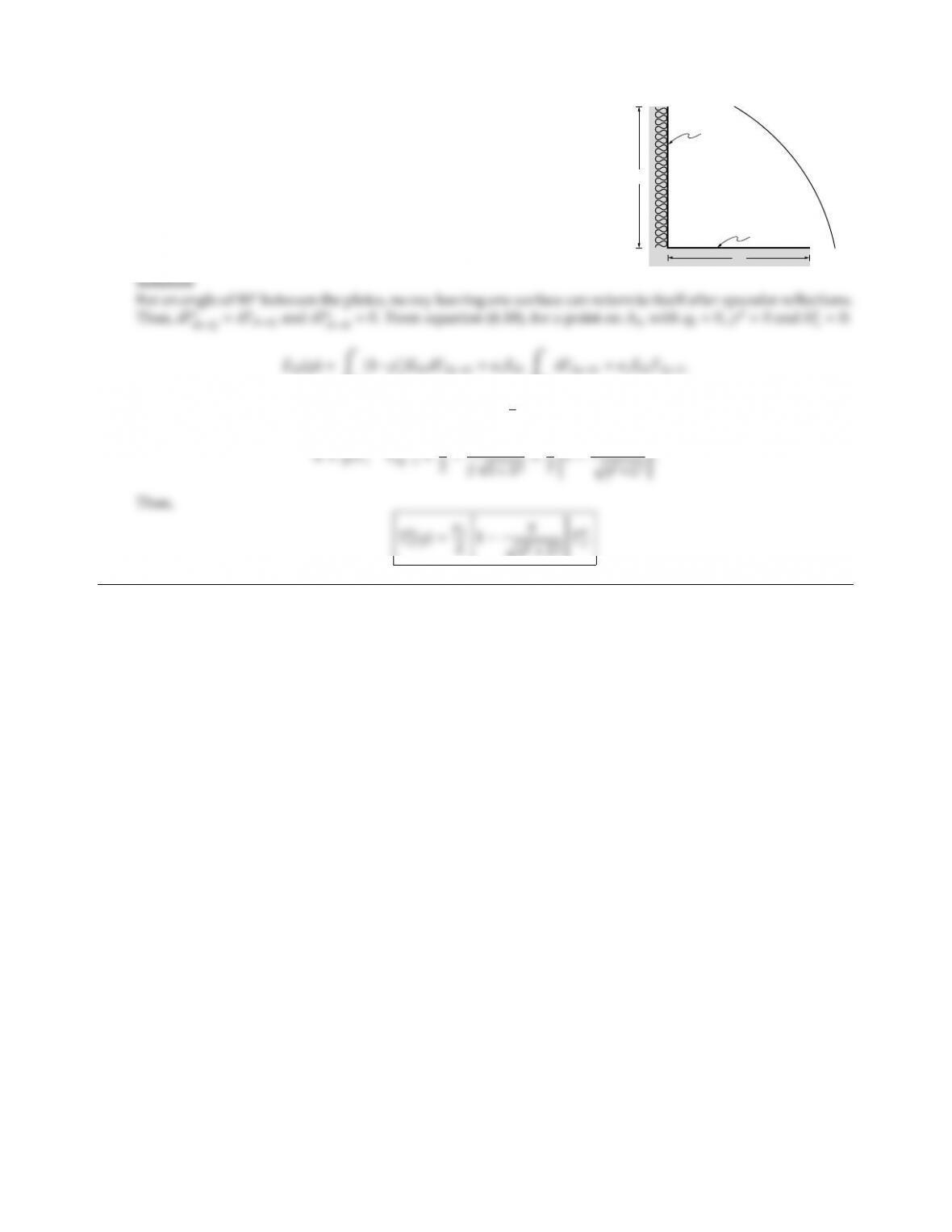CHAPTER 6 213
6.35
L
L
0 K
1, T1
2
An infinitely long cavity as shown is coated with gray, specular ma-
terials ǫ1and ǫ2(but the materials are diuse emitters). The vertical
surface is insulated, while the horizontal surface is at constant tem-
perature T1. The surroundings may be assumed to be black at 0 K.
Specify the variation of the temperature along the vertical plate.
1)Eb1dFdydx =ǫ1Eb1ZA1
Fdy1is easily evaluated, or can be found by setting φ=π
2in Configuration 23 in Appendix D:

## Trusted by Thousands ofStudents

Here are what students say about us.